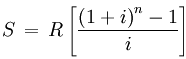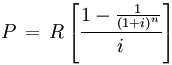SEARCH HOMEMath Central Quandaries & QueriesQuestion from Polly, a student: Susan contributed $500 every 6 months for 14 years into a RRSP earning interest @ 7.5% compounded semi-annually. Seven years after the last contribution Susan converted the RRSP into a RRIF which is to pay her equal 1/4 payments for 16 years. If the first payment is due 3 months after the conversion into the RRIF and the interest on the RRIF is 9% compounded 1/4, how much will Susan receive every 3 months?Hi Polly. Break it into pieces. Step 1: What is the value of the investment at the end of the 14 years of contributions? You can use this formula to help:where S is the value in 14 years, R is the payment size (500$), i is the interest per payment period (7.5% / 2 times each year = 0.0375) and n is the number of payments (14 x 2 = 28).

Step 2: What value does the RRSP grow to in the 7 years of dormancy?
You can use this formula to help:

A = P ( 1 + i )n

where A is the value after 7 years, P is the initial value (from step 1), i is the interest rate per compounding period (0.0375 again) and n is the number of compounding periods (7 times 2).

Step 3: Complete the problem by finding the quarterly payments needed to deplete the RRIF. A variant of the annuity formula from step 1 will do the job:Where P is the principle (the value A from step 2), R is what you need to find (so you need to re-arrange the equation a little), i is the interest rate per period (9% per year in quarters equals 0.0225), n is the number of periods (16 x 4).